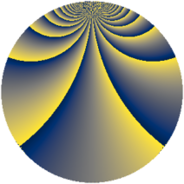# Properties

 Label 2736.3.heLevel $2736$ Weight $3$ Character orbit 2736.he Rep. character $\chi_{2736}(5,\cdot)$ Character field $\Q(\zeta_{36})$ Dimension $11472$ Sturm bound $1440$

# Related objects

## Defining parameters

 Level: $$N$$ $$=$$ $$2736 = 2^{4} \cdot 3^{2} \cdot 19$$ Weight: $$k$$ $$=$$ $$3$$ Character orbit: $$[\chi]$$ $$=$$ 2736.he (of order $$36$$ and degree $$12$$) Character conductor: $$\operatorname{cond}(\chi)$$ $$=$$ $$2736$$ Character field: $$\Q(\zeta_{36})$$ Sturm bound: $$1440$$

## Dimensions

The following table gives the dimensions of various subspaces of $$M_{3}(2736, [\chi])$$.

Total New Old
Modular forms 11568 11568 0
Cusp forms 11472 11472 0
Eisenstein series 96 96 0

## Trace form

 $$11472q - 18q^{2} - 12q^{3} - 6q^{4} - 18q^{5} - 12q^{6} + O(q^{10})$$ $$11472q - 18q^{2} - 12q^{3} - 6q^{4} - 18q^{5} - 12q^{6} - 24q^{10} - 6q^{12} - 6q^{13} - 18q^{14} - 24q^{15} - 6q^{16} - 24q^{18} - 24q^{19} - 36q^{20} - 66q^{21} - 6q^{22} - 12q^{24} + 432q^{26} - 6q^{27} + 72q^{28} - 18q^{29} - 594q^{30} - 24q^{31} - 18q^{32} - 24q^{33} - 6q^{34} - 450q^{35} - 12q^{36} - 48q^{37} - 18q^{38} - 6q^{40} - 528q^{42} - 6q^{43} + 594q^{44} - 6q^{45} - 12q^{46} - 36q^{47} - 12q^{48} + 39156q^{49} - 12q^{51} - 6q^{52} + 450q^{54} + 846q^{56} - 12q^{58} - 18q^{59} - 1104q^{60} - 6q^{61} - 72q^{62} - 612q^{63} - 12q^{64} - 1332q^{66} - 6q^{67} - 18q^{68} - 6q^{69} - 594q^{70} + 288q^{72} - 774q^{74} - 696q^{75} - 6q^{76} - 36q^{77} + 12q^{78} - 12q^{79} - 24q^{81} - 24q^{82} - 18q^{83} - 594q^{84} - 6q^{85} - 18q^{86} + 6q^{88} - 552q^{90} + 270q^{91} - 18q^{92} - 120q^{93} - 12q^{94} - 36q^{95} - 942q^{96} - 12q^{97} - 810q^{98} - 984q^{99} + O(q^{100})$$

## Decomposition of $$S_{3}^{\mathrm{new}}(2736, [\chi])$$ into newform subspaces

The newforms in this space have not yet been added to the LMFDB.